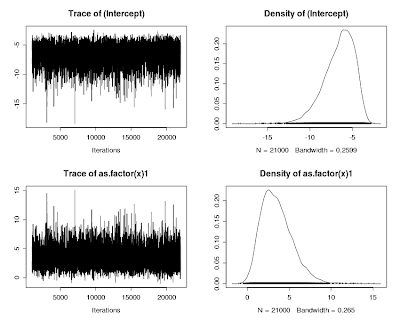## Monday, December 6, 2010

### Example 8.17: Logistic regression via MCMCIn examples 8.15 and 8.16 we considered Firth logistic regression and exact logistic regression as ways around the problem of separation, often encountered in logistic regression. (Re-cap: Separation happens when all the observations in a category share a result, or when a continuous covariate predicts the outcome too well. It results in a likelihood maximized when a parameter is extremely large, and causes trouble with ordinary maximum likelihood approached.) Another option is to use Bayesian methods. Here we focus on Markov chain Monte Carlo (MCMC) approaches to Bayesian analysis.

SAS

SAS access to MCMC for logistic regression is provided through the bayes statement in proc genmod. There are several default priors available. The normal prior is the most flexible (in the software), allowing different prior means and variances for the regression parameters. The prior is specified through a separate data set. We begin by setting up the data in the events/trials syntax. Then we define a fairly vague prior for the intercept and the effect of the covariate: uncorrelated, and each with a mean of zero and a variance of 1000 (or a precision of 0.001). Finally, we call proc genmod to implement the analysis.

`data testmcmc;  x=0; count=0; n=100; output;  x=1; count=5; n=100; output;run;data prior;  input _type_ \$ Intercept x;datalines;Var 1000 1000Mean 0 0 ;run;title "Bayes with normal prior";proc genmod descending data=testmcmc;  model count/n =  x / dist=binomial link=logit;  bayes seed=10231995 nbi=1000 nmc=21000    coeffprior=normal(input=prior) diagnostics=all    statistics=summary;run;`

In the forgoing, nbi is the length of the burn-in and nmc is the total number of Monte Carlo iterations. The remaining options define the prior and request certain output. The diagnostics=all option generates many results, including posterior autocorrelations, Gelman-Rubin, Geweke, Raftery-Lewis, and Heidelberger-Welch diagnostics. The summary statistics are presented below; the diagnostics are not especially encouraging.
`                      Posterior Summaries                                               Standard         Parameter           N        Mean    Deviation         Intercept       21000    -20.3301      10.3277         x               21000     17.2857      10.3368                      Posterior Summaries                                 Percentiles         Parameter         25%         50%         75%         Intercept    -27.6173    -18.5558    -11.9025         x              8.8534     15.5267     24.6024`

It seems that perhaps this prior is too vague. Perhaps we can make it a little more precise. A log odds ratio of 10 implies an odds ratio > 22,000, so perhaps we can accept a prior variance of 25, with about 95% of the prior weight between -10 and 10.
`data prior;  input _type_ \$ Intercept x;datalines;Var 25 25Mean 0 0 ;run;ods graphics on;title "Bayes with normal prior";proc genmod descending data=testmcmc;  model count/n =  x / dist=binomial link=logit;  bayes seed=10231995 nbi=1000 nmc=21000    coeffprior=normal(input=prior) diagnostics=all    statistics=(summary interval) plot=all;run;                      Posterior Summaries                                               Standard         Parameter           N        Mean    Deviation         Intercept       21000     -6.5924       1.7958         x               21000      3.5169       1.8431                      Posterior Summaries                                 Percentiles         Parameter         25%         50%         75%         Intercept     -7.6347     -6.3150     -5.2802         x              2.1790      3.2684      4.5929                      Posterior Intervals Parameter   Alpha   Equal-Tail Interval       HPD Interval Intercept   0.050   -10.8101    -3.8560   -10.2652    -3.5788 x           0.050     0.5981     7.7935     0.3997     7.4201`

These are more plausible values, and the diagnostics are more favorable.
In the above, we added the keyword interval to generate confidence regions, and used the ods graphics on statement to enable ODS graphics and the plot=all option to generate the graphical output shown above.

R
There are several packages in R that include MCMC approaches. Here we use the MCMCpack package, which include the MCMClogit() function. It appears not to accept the weights option mentioned previously, so we generate data at the observation level to begin. Then we run the MCMC.
`events.0=0   # for X = 0events.1=5   # for X = 1x = c(rep(0,100), rep(1,100))y = c(rep(0,100-events.0), rep(1,events.0),  rep(0, 100-events.1), rep(1, events.1))library(MCMCpack)logmcmc = MCMClogit(y~as.factor(x), burnin=1000, mcmc=21000, b0=0, B0=.04)`

The MCMClogit() accepts a formula object and allows the burn-in and number of Monte Carlo iterations to be specified. The prior mean b0 can be specified as a vector if different for each parameter, or as a scalar, as show. Similarly, the prior precision B0 can be a matrix or a scalar; if scalar, the parameters are uncorrelated in the prior.
`> summary(logmcmc)Iterations = 1001:22000Thinning interval = 1 Number of chains = 1 Sample size per chain = 21000 1. Empirical mean and standard deviation for each variable,   plus standard error of the mean:                Mean    SD Naive SE Time-series SE(Intercept)   -6.570 1.816  0.01253        0.03139as.factor(x)1  3.513 1.859  0.01283        0.033632. Quantiles for each variable:                  2.5%    25%    50%    75%  97.5%(Intercept)   -10.6591 -7.634 -6.299 -5.229 -3.831as.factor(x)1   0.6399  2.147  3.292  4.599  7.698plot(logmcmc)`

The result of the plot() is shown below. These results and those from SAS are reassuringly similar. Many diagnostics are available through the coda package. The codamenu() function allows simple menu-based access to its tools.